# Markov Random Field Optimisation

Peter Orchard

### Markov Random Fields

A Markov Random Field (MRF) is a graphical model of a joint probability distribution. It consists of an undirected graph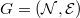in which the nodes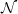represent random variables. Let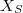be the set of random variables associated with the set of nodes S. Then, the edges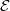encode conditional independence relationships via the following rule: given disjoint subsets of nodes A, B, and C,is conditionally independent of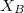given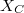if there is no path from any node in A to any node in B that doesn't pass through a node of C. We write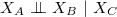. If such a path does exist, the subsets are dependent.

The neighbour set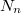of a node n is defined to be the set of nodes that are connected to n via edges in the graph: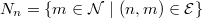Given its neighbour set, a node n is independent of all other nodes in the graph. Therefore, we may write the following for the conditional probability of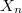: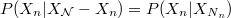This is the Markov property, and is where the model gets its name. The following diagram illustrates the concept: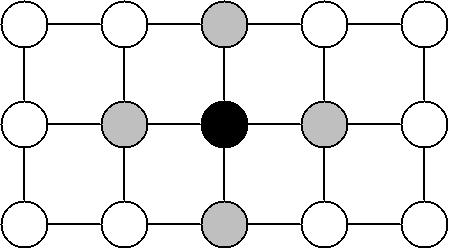Figure 1: Given the grey nodes, the black node is conditionally independent of all other nodes.

From this point, "node" and "variable" shall be used interchangeably. x shall refer to a particular configuration of the set of the random variables. A subscript will denote a particular node or subset of nodes.

The Markov property tells us that the joint distribution of X is determined entirely by the local conditional distributions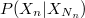. But it is not clear how to actually construct the global joint distribution from these local functions. In order to do this, we need to look at Gibbs distributions.

A Gibbs distribution on the graph G takes the form: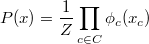where the product is over all maximal cliques in the graph. A clique is a subset of nodes in which every node is connected to every other node. A maximal clique is a clique which cannot be extended by the addition of another node. Z is called the partition function, and takes the form: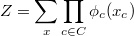Theare usually written: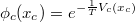T is called the temperature, and is often taken to be 1. So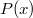has the alternate form: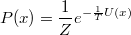where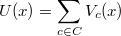is called the energy. Either the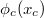or themay be referred to as clique potentials.

The Hammersley-Clifford theorem states that the joint probability distribution of any MRF can be written as a Gibbs distribution, and furthermore that for any Gibbs distribution there exists an MRF for which it is the joint. That is to say, Hammersley-Clifford establishes the equality of the MRF and Gibbs models. This solves the problem of how to specify the joint distribution of an MRF in terms of local functions: it can now be specified by defining the potential on every maximal clique.

### Optimisation

An optimisation problem is one that involves finding the extremum of a quantity or function. Such problems often arise as a result of a source of uncertainty that precludes the possibility of an exact solution.

Optimisation in an MRF problem involves finding the maximum of the joint probability over the graph, usually with some of the variables given by some observed data. Equivalently, as can be seen from the equations above, this can be done by minimising the total energy, which in turn requires the simultaneous minimisation of all the clique potentials.

Techniques for minimisation of the MRF potentials are plentiful. Many of them are also applicable to optimisation problems other than MRF. For example, gradient descent methods are well-known techniques for finding local minima, while the closely-related method of simulated annealing attempts to find a global minimum.

An example of a technique invented specifically for MRF optimisation is Iterated Conditional Modes (ICM). This simple algorithm proceeds first by choosing an initial configuration for the variables. Then, it iterates over each node in the graph and calculates the value that minimises the energy given the current values for all the variables in its neighbourhood. At the end of an iteration, the new values for each variable become the current values, and the next iteration begins. The algorithm is guaranteed to converge, and may be terminated according to a chosen criterion of convergence. An example of this technique in action can be seen below.

### MRF Applications To Vision

Problems in computer vision usually involve noise, and so exact solutions are most often impossible. Additionally, the latent variables of interest often have the Markov property. For example, the pixel values in an image usually depend most strongly on those in the immediate vicinity, and have only weak correlations with those further away. Therefore, vision problems are well suited to the MRF optimisation technique. Some examples of vision problems to which MRFs have been applied are:

1. Image restoration
2. Image reconstruction
3. Segmentation
4. Edge detection
In the first 3 of these, there is a latent random variable for each pixel. The variables range over intensity values in 1 and 2, while in 3 the variables take on segment identifiers. In problem 4, the variables correspond to pairs of neighbouring pixels, and their values are binary, indicating the presence or absence of an edge. In all cases, the neighbourhood concept of the MRF maps to the geometric neighbourhood of the image. That is to say, the edges in the MRF graph will connect geometrically close pixels or edges. The appropriate size of the neighbourhood depends on the problem at hand: a larger neighbourhood has more modelling power at the expense of greater computational demand during optimisation.

The following diagram illustrates the structure of an MRF that could be used for image restoration. It also shows how "variables" corresponding to known data can be incorporated into the model. The white nodes represent the unknown true pixel values, and the grey nodes represent the noisy pixel data. Notice that the latent variables are connected to their neighbouring pixels, but the data points are connected only to one latent variable. This models our belief that the estimated pixel values should depend on both the noisy data and the estimated neighbouring pixel values.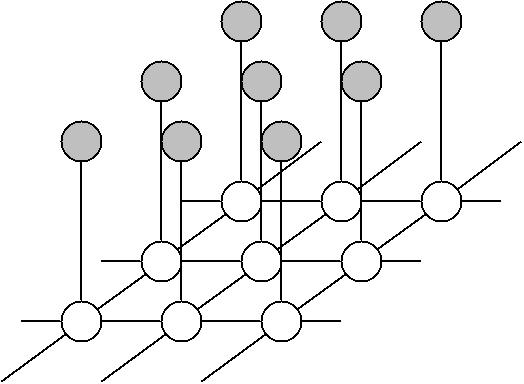Figure 2: Section of an MRF for an image restoration problem.

Having constructed an MRF, the clique potentials must be defined. This encodes the relationship between variables, and so this is where we get to specify what we want from the solution. Finding an appropriate energy function and selecting the parameters that give an acceptable solution requires insight, as well as trial and error. However, there are many often-used, standard energy functions for different types of problem. For example, if the graph of figure 2 were used for image restoration, clique potentials might take the form: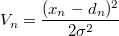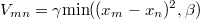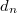is the observed value at data node n. The first equation is the potential for the cliques containing one data node and one latent node. It expresses a belief that the image is corrupted by Gaussian noise of variance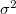. The second equation is the potential for cliques containing two latent nodes. It punishes differences between neighbouring nodes, but only up to a maximum of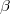. This represents a statement that most regions are smooth, but that edges are expected and so should not be punished too harshly. The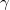weights the importance of the second type of potential relative to the first.

### Example

Here is an example of the results from an application of MRFs to an image restoration problem. The structure of the MRF was as in figure 2, and the potentials were those stated above. The original image looked like this:Figure 3: The original image.

Then, this matlab code was used to add Gaussian noise to the image with covariance of 100, resulting in figure 4.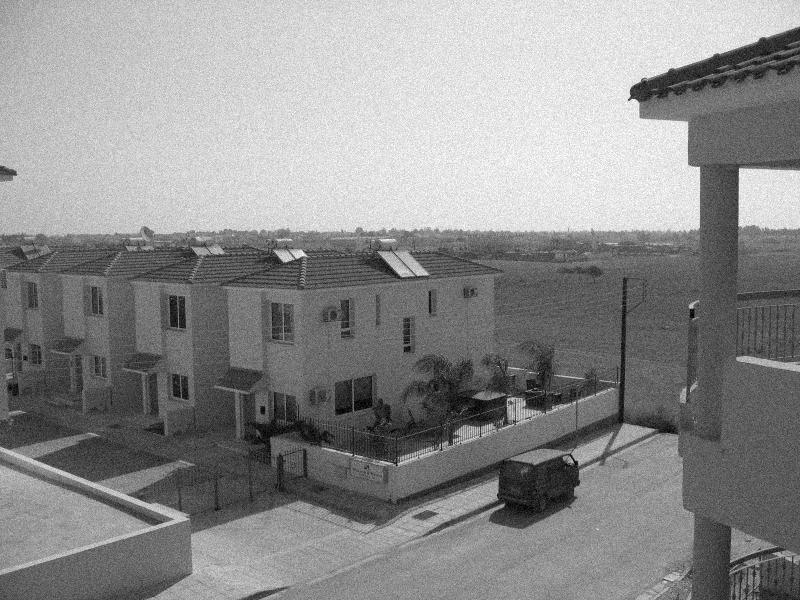Figure 4: The image with added Gaussian noise.

Finally, this matlab code was used to smooth the image. The parameters to restore_image were:

• max_diff = 200
• weight_diff = 0.02
• iterations = 10
The code uses a simple implementation of the ICM algorithm. It is intended as a clear example of an MRF optimisation method, and so is not at all optimised for efficiency. The result was figure 5.Figure 5: The restored image.

A comparison of figures 4 and 5 shows that this restoration method has done quite a good job of smoothing the noise from surfaces of constant or slowly-varying intensity such as the road, the sides of buildings, and the sky. It does not do so well with thin, sharp features; for example, the telephone cables are almost completely removed. This is due to the energy function punishing local differences in pixel values.

### References

1. C Bishop. Pattern Recognition and Machine Learning Springer, 383-393, 2006
2. S Geman and D Geman. Stochastic Relaxation, Gibbs Distributions, and the Bayesian Restoration of Images IEEE Transactions on Pattern Analysis and Machine Intelligence, 6(6):721-741, 1984
3. R Kindermann and J L Snell. Markov Random Fields and Their Applications American Mathematical Society, 1980
4. S Li. Markov Random Field Modeling in Computer Vision Springer-Verlag, 1995
5. P Perez. Markov Random Fields and Images CWI Quarterly, 11(4):413-437, 1998
6. G Winkler. Image Analysis, Random Fields and Dynamic Monte Carlo Methods Springer-Verlag, 1995